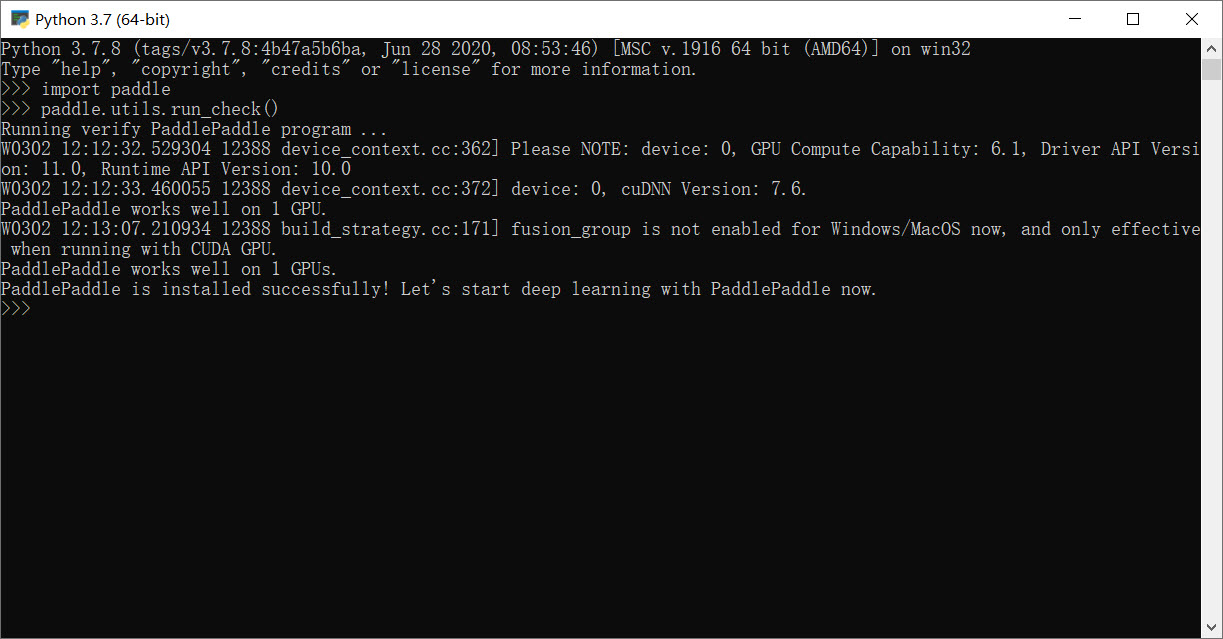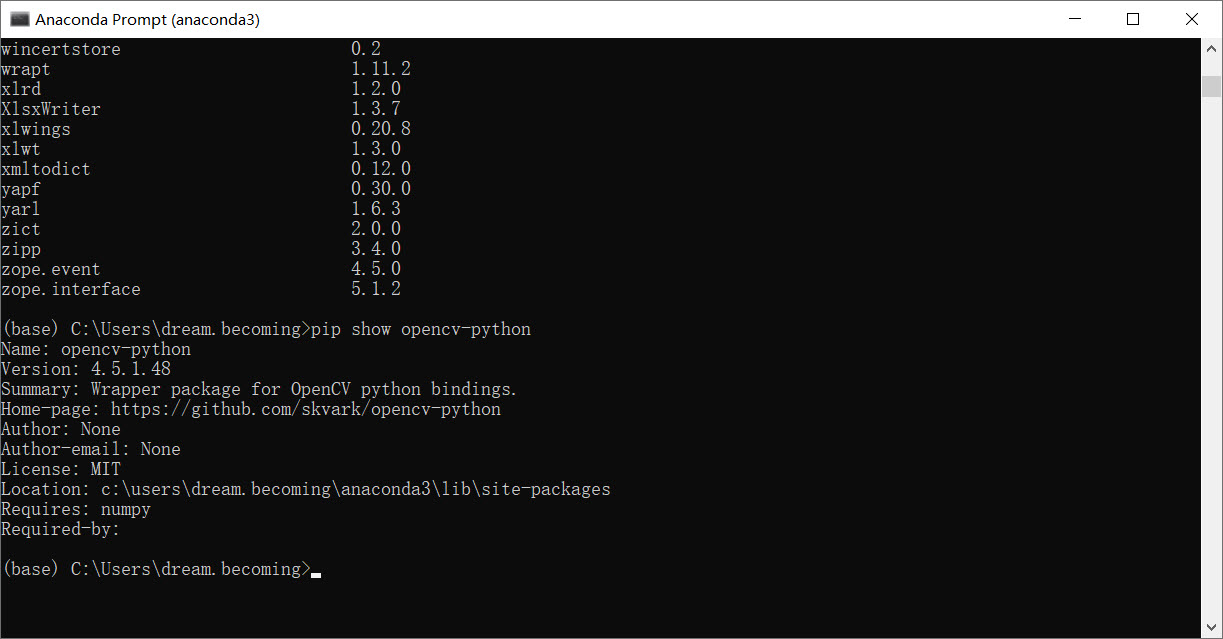# 课节1：课前资料

## python 的__call__()方法

class A:
def __init__(self):
print("__init__ ")
print(self)
super(A, self).__init__()

def __new__(cls):
print("__new__ ")
self = super(A, cls).__new__(cls)
print(self)
return self

def __call__(self):  # 可以定义任意参数
print('__call__ ')

A()


__new__
<__main__.A object at 0x1007a95f8>
__init__
<__main__.A object at 0x1007a95f8>

# a是实例对象，同时还是可调用对象
a = A()
print(callable(a))  # True

• new 方法的返回类的实例对象，传递给 init 方法中定义的 self 参数
• init()的作用是初始化某个类的一个实例,返回 None 值
• call()的作用是使实例能够像函数一样被调用

1. 明确指定：
class  C(P):
def __init__(self):
P.__init__(self)
print 'calling Cs construtor'

1. 使用super()方法 ：
class  C(P):
def __init__(self):
super(C,self).__init__()
print 'calling Cs construtor'

• 单继承结果一样，多继承super更好。

• 对于定义的类，在Python中会创建一个MRO(Method Resolution Order)列表，它代表了类继承的顺序。MRO的查找顺序是按广度优先

• super().method()是调用父类中的方法，这个搜索顺序当然是按照MRO从前向后开始进行的。

super([type][, object-or-type])

根据官方文档，super函数返回一个委托类type的父类或者兄弟类方法调用的代理对象。super函数用来调用已经再子类中重写过的父类方法。

常见的是直接调用super(),这其实是super(type, obj)的简写方式，将当前的类传入type参数，同时将实例对象传入type-or-object参数，这两个实参必须确保isinstance(obj, type)为True。

使用该方法调用的super函数返回的代理类是obj所属类的MRO中，排在type之后的下一个父类。

继承结构：
Base
/  \
/    \
A      B
\    /
\  /
C

• 不使用super:
class Base(object):
def __init__(self):
print ("enter Base")
print ("leave Base")

class A(Base):
def __init__(self):
print ("enter A")
Base().__init__()
print ("leave A")

class B(Base):
def __init__(self):
print ("enter B")
Base().__init__()
print ("leave B")

class C(A, B):
def __init__(self):
print ("enter C")
A().__init__()
B().__init__()
print ("leave C")

print (C.mro())
C()


[<class '__main__.C'>, <class '__main__.A'>, <class '__main__.B'>, <class '__main__.Base'>, <class 'object'>]
enter C
enter A
enter Base
leave Base
enter Base
leave Base
leave A
enter A
enter Base
leave Base
enter Base
leave Base
leave A
enter B
enter Base
leave Base
enter Base
leave Base
leave B
enter B
enter Base
leave Base
enter Base
leave Base
leave B
leave C
<__main__.C at 0x7f734302db10>

• 使用super:
class Base(object):
def __init__(self):
print ("enter Base")
print ("leave Base")

class A(Base):
def __init__(self):
print ("enter A")
super(A, self).__init__()
print ("leave A")

class B(Base):
def __init__(self):
print ("enter B")
super(B, self).__init__()
print ("leave B")

class C(A, B):
def __init__(self):
print ("enter C")
super(C, self).__init__()
print ("leave C")

print (C.mro())
C()


[<class '__main__.C'>, <class '__main__.A'>, <class '__main__.B'>, <class '__main__.Base'>, <class 'object'>]
enter C
enter A
enter B
enter Base
leave Base
leave B
leave A
leave C
<__main__.C at 0x7f734304e090>

• isinstance() 函数来判断一个对象是否是一个已知的类型，类似 type()。

• isinstance() 与 type() 区别：

type() 不会认为子类是一种父类类型，不考虑继承关系。

isinstance() 会认为子类是一种父类类型，考虑继承关系。

• isinstance(object, classinfo)
object – 实例对象。
classinfo – 可以是直接或间接类名、基本类型或者由它们组成的元组。其中基本类型：int，float，bool，complex，str(字符串)，list，dict(字典)，set，tuple。

class A:
pass

class B(A):
pass

isinstance(A(), A)    # returns True
type(A()) == A        # returns True
isinstance(B(), A)    # returns True
type(B()) == A        # returns False

print('A\t:',A)
print('A()\t:',A())
print('type(A())\t:',type(A()))
print('type(A())== A\t:', type(A()) == A  )
print('判断A()实例对象，A类名 的类型是否相同：',isinstance(A(), A))


输出：

A	: <class '__main__.A'>
A()	: <__main__.A object at 0x7f7342ff3350>
type(A())	: <class '__main__.A'>
type(A())== A	: True
判断A()实例对象，A类名 的类型是否相同： True


## 图像基础知识

• 在计算机中, 图像是由一个个像素点组成，像素点就是颜色点，而颜色最简单的方式就是用 RGB 或 RGBA 表示。如果有A通道就表明这个图像可以有透明效果。
R,G,B 每个分量一般是用一个字节(8位)来表示，所以RGB图中每个像素大小就是3 * 8=24位图, 而RGBA图中每个像素大小是4 * 8=32位。
• 图像是二维数据，数据在内存中只能一维存储，二维转一维有不同的对应方式。比较常见的只有两种方式: 按像素“行排列” 从上往下 或者 从下往上。
• 一般只会有RGB,BGR, RGBA, RGBA, BGRA这几种排列据。 绝大多数图形库或环境是BGR/BGRA排列，cocoa中的NSImage或UIImage是RGBA排列。

1. 调用函数计算：byteNum = ceil(bm.bmWidth / 32) * 4。图片实际存储宽度 除以32 再向上取整，32位相当于4字节，所以 乘以4 得出行字节数。
1. 使用位运算：byteNum = ((bm.bmWidth + 31) & ~31) >> 3。图片实际存储宽度 加了31 与 取反后的31 按位与之后，后面5个位都置为0，这相当于向下取32的最大倍数；>>3右位移3位，即除以8，得出行字节数。
#图像宽为5像素，不做32位对齐的话，其行位数为24*5=120，120不是32的倍数。是32整数倍并且刚好比120大的数是128
print('二进制31\t',bin(31))
print('二进制~31\t',bin(~31))
print('二进制32\t',bin(32))

print('\n')
print('二进制120+31=151\t',bin(151))
print('二进制~31\t',bin(~31))
print('十进制(151 & ~31):\t{a}\n二进制(151 & ~31):\t{b} '.format(a=(151 & ~31),b=bin(151 & ~31)))

二进制31	 0b11111



## 图片格式

• BMP格式

bmp格式没有压缩像素格式，存储在文件中时先有文件头、再图像头、后面就都是像素数据了，上下颠倒存储。 用windows自带的mspaint工具保存bmp格式时，可以发现有四种bmp可供选择：

• 单色: 一个像素只占一位，要么是0，要么是1，所以只能存储黑白信息
• 16色位图: 一个像素4位，有16种颜色可选
• 256色位图: 一个像素8位，有256种颜色可选
• 24位位图: 就是图(1)所示的位图，颜色可有2^24种可选，对于人眼来说完全足够了。
• BJPEG格式

• jpeg是有损压缩格式, 将像素信息用jpeg保存成文件再读取出来，其中某些像素值会有少许变化。在保存时有个质量参数可在[0,100]之间选择，参数越大图片就越保真，但图片的体积也就越大。一般情况下选择70或80就足够了。
• jpeg没有透明信息。
• jpeg比较适合用来存储相机拍出来的照片，这类图像用jpeg压缩后的体积比较小。其使用的具体算法核心是离散余弦变换、Huffman编码、算术编码等技术
• PNG格式

• png是一种无损压缩格式， 压缩大概是用行程编码算法。
• png可以有透明效果。
• png比较适合适量图,几何图。
• GIF格式

• WebP编码

Webp是一种高效的图像编码方式，由谷歌推出，开源免费。其图像压缩效率相比jpg可以提升一倍性能。一般保存需要设置压缩因子。

## 常用库

• Numpy
NumPy(Numerical Python) 是 Python 语言的一个扩展程序库，支持大量的维度数组与矩阵运算，此外也针对数组运算提供大量的数学函数库。
导入：import numpy as np
创建数组：
• numpy.array(object, dtype = None, copy = True, order = None, subok = False, ndmin = 0)
• arange([start,] stop[, step,], dtype=None)，返回类型：ndarray，N 维数组对象 ndarray。
• range()中的步长不能为小数，但是np.arange()中的步长可以为小数
import numpy as np
print(np.arange(3))
print(type(np.arange(3)))

print('\n')
print(np.array([1,2,3])  )
print(type(np.array([1,2,3]) ))

输出：
[0 1 2]
<class 'numpy.ndarray'>

[1 2 3]
<class 'numpy.ndarray'>


python array=np.array([[1,2,3],[4,5,6],[7,8,9],[10,11,12]]) print(array) #数组维度 print(array.ndim) #数组形状 print(array.shape) #数组元素个数 print(array.size) #数组元素类型 print(array.dtype)

python [[ 1 2 3] [ 4 5 6] [ 7 8 9] [10 11 12]] 2 (4, 3) 12 int64

arr：要修改形状的数组 。
newshape：整数或者整数数组，新的形状应当兼容原有形状 。
order：‘C’ – 按行，‘F’ – 按列，‘A’ – 原顺序，‘k’ – 元素在内存中的出现顺序。

* 矩阵乘法 (dot) ，对应分量相乘 (multiply或 *)

• CV2
读取BGR，通道HWC，范围[0,255] ，类型uint8; 图像类型numpy.ndarray；

• PIL，Pillow, Pillow-SIMD
读取RGB，通道HWC，范围[0,255]，类型uint8；图像类型PngImageFile （np.array, Image.fromarray直接与numpy互相转换） 有.mode方法—rgb信息

• Matplotlib
读取RGB，通道HWC，范围[0,1] ，类型float；图像类型numpy.ndarray

• Skimage
读取RGB，通道HWC，范围[0,255]，类型uint8；图像类型numpy.ndarray 有.mode方法—rgb信息

## 常见操作

### 读取

# cv2 默认
# 彩色图，默认值(1)，灰度图(0)，包含透明通道的彩色图(-1)
img = cv2.imread('examples.png')
img_gray = cv2.imread('examples.png', 0)
img_unchanged = cv2.imread('examples.png', -1)

PIL，Pillow, Pillow-SIMD
img = Image.open('examples.png')

Matplotlib
img = plt.imread('examples.png')


### 显示

# Matplotlib
img = plt.imread('examples.png')
plt.imshow(img)
plt.show()

# CV2
img = cv2.imread('examples.png')
plt.imshow(img[..., -1::-1]) # 因为opencv读取进来的是bgr顺序，而imshow需要的是rgb顺序，因此需要先反过来,也可以plt.imshow(img[:,:,::-1])
plt.show()

# PIL
#可直接打开
plt.imshow(Image.open('examples.png')) # 实际上plt.imshow可以直接显示PIL格式图像
plt.show()

# 转换为需要的numpy格式打开
img_gray = img.convert('L') #转换成灰度图像
img = np.array(img)
img_gray = np.array(img_gray)
plt.imshow(img) # or plt.imshow(img / 255.0)5
plt.show()
plt.imshow(img_gray, cmap=plt.gray()) # 显示灰度图要设置cmap参数
plt.show()

• plt.imshow()函数负责对图像进行处理，并显示其格式，但是不能显示。其后跟着plt.show()才能显示出来。
• cmap将标量数据映射到色彩图

### 转换

a是rgb图像，那么

a[::-1]，a[:,::-1]，a[:,:,::-1]分别是X轴的镜像，Y轴的镜像，BGR转换为RGB；

# opencv相关图像操作；
img_gray = cv2.cvtColor(img,cv2.COLOR_BGR2GRAY) # BGR转灰度
img_bgr = cv2.cvtColor(img_gray,cv2.COLOR_GRAY2BGR) # 灰度转BRG
img_rgb = cv2.cvtColor(img_gray,cv2.COLOR_GRAY2RGB) # 灰度转RGB

b,g,r = cv2.split(img) #bgr图像分离三个通道
img2 = cv2.merge([r,g,b]) #merge成rgb图像

# PIL相关图像操作：
img = Image.open('examples.png')
img_gray = image.convert(‘L’)
img_color = img_gray.convert(‘RGB’)

#PIL与numpy格式转换操作：
numpy.asarray()
Image.fromarray()


PIL类型，尺寸信息，通过.size方法，得到WH；

Numpy类型，通过shape方法，得到HWC

### 保存

# PIL
# 直接save方法

img = Image.open('examples.png')
img.save('examples2.png')
img_gray = img.convert('L')
img_gray.save('examples_gray.png') # 不管是灰度还是彩色，直接用save函数保存就可以，但注意，只有PIL格式的图片能够用save函数

#cv2.imwrite

import cv2
img = cv2.imread('examples.png') # 这是BGR图片
cv2.imwrite('examples2.png', img) # 这里也应该用BGR图片保存，这里要非常注意，因为用pylab或PIL读入的图片都是RGB的，如果要用opencv存图片就必须做一个转换
img_gray = cv2.cvtColor(img,cv2.COLOR_BGR2GRAY)
cv2.imwrite('examples_gray.png', img_gray)


## 预习作业

1.本地安装PaddlePaddle，截图并上传

 提示：使用 python 进入python解释器，输入import paddle ，再输入 paddle.utils.run_check()。2.本地安装open-cv-python，截图上传

 终端下输入： pip show opencv-python03-072002©️2020 CSDN 皮肤主题: 游动-白 设计师:白松林